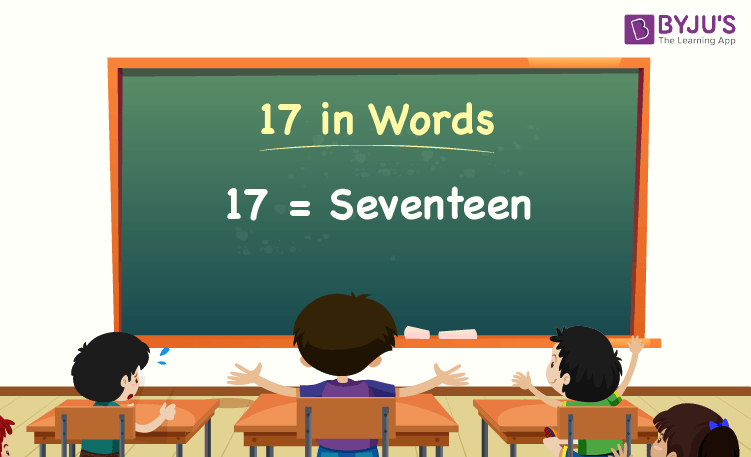# 17 in Words

17 in words is Seventeen. For example, if you earned Rs. 17 in a day, you can write, “I have earned Rs. Seventeen in a day”. In general, the number name of any number can be written using the ones, tens, hundreds, and thousands place of a number. Thus, the place value chart is useful for writing the number 17 in words.

 17 in Words: Seventeen

In this article, we will learn how to write 17 in words, and look at the solved examples in detail.

## How to Write 17 in Words?To write the number name of 17, first, we have to determine the place value of each digit of the given number 17. Hence, for the number 17,

1’s place of 17 is 7

10’s place of 17 is 1.

Since 17 is a two-digit number, there are no hundreds and thousands.

The following chart represents the place value of the number 17:

 Tens Ones 1 7

Therefore, the number 17 in words is Seventeen.

### Examples

Example 1:

Find the value of 11 + 6. Describe the value in words.

Solution:

Given expression: 11 + 6

⇒ 11 + 6 = 17

So, the value of 11 + 6 is 17.

Hence, 17 in words is seventeen.

Example 2:

Express the value of seventeen plus hundred in words.

Solution:

Seventeen = 17

Hundred = 100

Seventeen plus hundred = 17 + 100 = 117

Hence, the value of seventeen plus hundred is one hundred seventeen in words.

Stay tuned to BYJU’S – The Learning App, and download the app to learn all Maths concepts quickly by exploring more learning videos.

## Frequently Asked Questions on 17 in Words

Q1

### Write 17 in words.

17 in words is seventeen.

Q2

### Express the value 20 – 3 in words.

Simplifying 20 – 3, we get 17. Hence, 17 in words is seventeen.

Q3

### How to write seventeen in numbers?

Seventeen in numbers is 17.

Q4

### Is the number 17 an odd number?

Yes, the number 17 is an odd number.

Q5

### Is 17 a composite number?

No, 17 is not a composite number.